Inverse Functions

A functionis one-to-one (1-1) if it does not assign the same value to two different elements of its domain:

If, then.

If f is a 1-1 function, then it has an inverse functiondefined by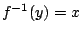iff, for allin the range of f.

The domain ofis the range of, and the range ofis the domain of.

To find a formula for, we can

1. Set.

2. Solve for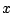in terms of, if possible.

3. Set.

[Another common way to do this is to

1. Set, and then interchangeand.

2. Solve forin terms of, if possible.

3. Set.]

Ex 1 Show whether or not the function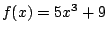is one-to-one.

Sol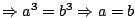, sois a 1-1 function.

Ex 2 Show whether or not the functionis one-to-one.

Sol Setting, for example, and solving gives that; sois not a 1-1 function.

Ex 3 If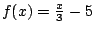, find a formula forSol Let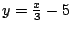. Then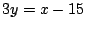, so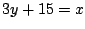or. Thus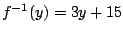, so.

Pr 1 If, find a formula for.

Pr 2 If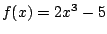, find a formula for.

Pr 3 If, find a formula forand find the domain for.

Pr 4 If, find a formula for.

Pr 5 Show whether or not the function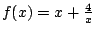has an inverse.

Pr 6 Letfor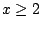. Find a formula forand find the domain for.

Pr 7 If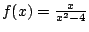for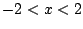, find.

Pr 8 If, find a formula forand find the domain for.